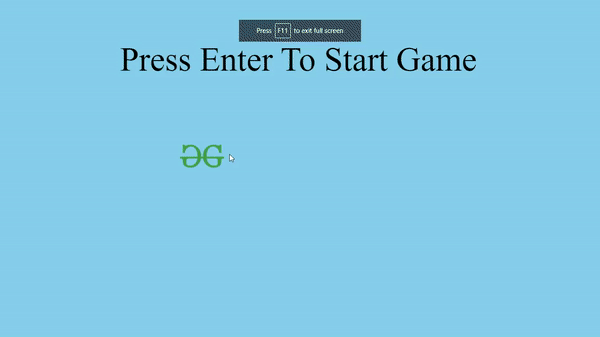GeeksforGeeks App
Open AppBrowser
Continue

# Flappy Bird Game in JavaScript

Flappy Bird is an endless game that involves a bird that the player can control. The player has to save the bird from colliding with the hurdles like pipes. Each time the bird passes through the pipes, the score gets incremented by one. The game ends when the bird collides with the pipes or falls down due to gravity. The sections below describe the steps that have to be taken for building this game.

HTML Section: In this section, the elements of the game are created and loaded. The images for the background, bird, hurdles and the score elements are selected. Next, we create and link the style.css and index.js file.

## HTML

 ```<``html``>`` ` `<``head``>``    ``<``link` `rel``=``"stylesheet"` `href``=``"style.css"``>```` ` `<``body``>``    ``<``div` `class``=``"background"``>``    ``<``img` `class``=``"bird"` `src``=``"logo.png"` `alt``=``"bird-img"``>``    ``<``div` `class``=``"message"``>``        ``Press Enter To Start Game``    ````    ``<``div` `class``=``"score"``>``        ``<``span` `class``=``"score_title"``>``        ``<``span` `class``=``"score_val"``>``    ````    ``<``script` `src``=``"gfg.js"``>```` ` ``

CSS Section: In this section, the size, position and style of the game objects are modified according to need.

## CSS

 `* {``    ``margin``: ``0``;``    ``padding``: ``0``;``    ``box-sizing: border-box;``}`` ` `body {``    ``height``: ``100``vh;``    ``width``: ``100``vw;``}`` ` `.background {``    ``height``: ``100``vh;``    ``width``: ``100``vw;``    ``background-color``: skyblue;``}`` ` `.bird {``    ``height``: ``100px``;``    ``width``: ``160px``;``    ``position``: ``fixed``;``    ``top``: ``40``vh;``    ``left``: ``30``vw;``    ``z-index``: ``100``;``}`` ` `.pipe_sprite {``    ``position``: ``fixed``;``    ``top``: ``40``vh;``    ``left``: ``100``vw;``    ``height``: ``70``vh;``    ``width``: ``6``vw;``    ``background-color``: ``green``;``}`` ` `.message {``    ``position``: ``fixed``;``    ``z-index``: ``10``;``    ``height``: ``10``vh;``    ``font-size``: ``10``vh;``    ``font-weight``: ``100``;``    ``color``: ``black``;``    ``top``: ``12``vh;``    ``left``: ``20``vw;``    ``text-align``: ``center``;``}`` ` `.score {``    ``position``: ``fixed``;``    ``z-index``: ``10``;``    ``height``: ``10``vh;``    ``font-size``: ``10``vh;``    ``font-weight``: ``100``;``    ``color``: goldenrod;``    ``top``: ``0``;``    ``left``: ``0``;``}`` ` `.score_val {``    ``color``: gold;``}`

JavaScript Section: This section contains the part of the code that controls the game state and the moving objects. The following steps have to be followed in this section.

• Get a reference to bird and background image in JavaScript file.
• Set some values for background scrolling speed, the flying speed of the bird, and gravity.
• Create the infinite scrolling background. A guide for doing this can be read from this link.
• Add an event listener to listen for the “enter” keypress to change the game state to the play state and apply gravity to the bird by decreasing the gravity value from the bird’s y-coordinate every frame.
• Generate hurdles (pipes) at the end of the view width so that they are not visible initially, but as the background moves, decrease the pipe’s x-coordinate by the background scrolling value so that it looks like the bird is moving.
• Apply collision with ground and pipes and if the bird collides then change the game state to end state and show a message to restart the game.
• Increment score value after every successful navigation between the pipes.

## JavaScript

 `// Background scrolling speed``let move_speed = 3;``   ` `// Gravity constant value``let gravity = 0.5;``   ` `// Getting reference to the bird element``let bird = document.querySelector(``'.bird'``);``   ` `// Getting bird element properties``let bird_props = bird.getBoundingClientRect();``let background =``    ``document.querySelector(``'.background'``)``            ``.getBoundingClientRect();``   ` `// Getting reference to the score element``let score_val =``    ``document.querySelector(``'.score_val'``);``let message =``    ``document.querySelector(``'.message'``);``let score_title =``    ``document.querySelector(``'.score_title'``);``   ` `// Setting initial game state to start``let game_state = ``'Start'``;``   ` `// Add an eventlistener for key presses``document.addEventListener(``'keydown'``, (e) => {``   ` `  ``// Start the game if enter key is pressed``  ``if` `(e.key == ``'Enter'` `&&``      ``game_state != ``'Play'``) {``    ``document.querySelectorAll(``'.pipe_sprite'``)``              ``.forEach((e) => {``      ``e.remove();``    ``});``    ``bird.style.top = ``'40vh'``;``    ``game_state = ``'Play'``;``    ``message.innerHTML = ``''``;``    ``score_title.innerHTML = ``'Score : '``;``    ``score_val.innerHTML = ``'0'``;``    ``play();``  ``}``});``function` `play() {``  ``function` `move() {``     ` `    ``// Detect if game has ended``    ``if` `(game_state != ``'Play'``) ``return``;``     ` `    ``// Getting reference to all the pipe elements``    ``let pipe_sprite = document.querySelectorAll(``'.pipe_sprite'``);``    ``pipe_sprite.forEach((element) => {``       ` `      ``let pipe_sprite_props = element.getBoundingClientRect();``      ``bird_props = bird.getBoundingClientRect();``       ` `      ``// Delete the pipes if they have moved out``      ``// of the screen hence saving memory``      ``if` `(pipe_sprite_props.right <= 0) {``        ``element.remove();``      ``} ``else` `{``        ``// Collision detection with bird and pipes``        ``if` `(``          ``bird_props.left < pipe_sprite_props.left +``          ``pipe_sprite_props.width &&``          ``bird_props.left +``          ``bird_props.width > pipe_sprite_props.left &&``          ``bird_props.top < pipe_sprite_props.top +``          ``pipe_sprite_props.height &&``          ``bird_props.top +``          ``bird_props.height > pipe_sprite_props.top``        ``) {``           ` `          ``// Change game state and end the game``          ``// if collision occurs``          ``game_state = ``'End'``;``          ``message.innerHTML = ``'Press Enter To Restart'``;``          ``message.style.left = ``'28vw'``;``          ``return``;``        ``} ``else` `{``          ``// Increase the score if player``          ``// has the successfully dodged the ``          ``if` `(``            ``pipe_sprite_props.right < bird_props.left &&``            ``pipe_sprite_props.right + ``            ``move_speed >= bird_props.left &&``            ``element.increase_score == ``'1'``          ``) {``            ``score_val.innerHTML = +score_val.innerHTML + 1;``          ``}``          ``element.style.left = ``            ``pipe_sprite_props.left - move_speed + ``'px'``;``        ``}``      ``}``    ``});`` ` `    ``requestAnimationFrame(move);``  ``}``  ``requestAnimationFrame(move);`` ` `  ``let bird_dy = 0;``  ``function` `apply_gravity() {``    ``if` `(game_state != ``'Play'``) ``return``;``    ``bird_dy = bird_dy + gravity;``    ``document.addEventListener(``'keydown'``, (e) => {``      ``if` `(e.key == ``'ArrowUp'` `|| e.key == ``' '``) {``        ``bird_dy = -7.6;``      ``}``    ``});`` ` `    ``// Collision detection with bird and``    ``// window top and bottom`` ` `    ``if` `(bird_props.top <= 0 ||``        ``bird_props.bottom >= background.bottom) {``      ``game_state = ``'End'``;``      ``message.innerHTML = ``'Press Enter To Restart'``;``      ``message.style.left = ``'28vw'``;``      ``return``;``    ``}``    ``bird.style.top = bird_props.top + bird_dy + ``'px'``;``    ``bird_props = bird.getBoundingClientRect();``    ``requestAnimationFrame(apply_gravity);``  ``}``  ``requestAnimationFrame(apply_gravity);`` ` `  ``let pipe_seperation = 0;``   ` `  ``// Constant value for the gap between two pipes``  ``let pipe_gap = 35;``  ``function` `create_pipe() {``    ``if` `(game_state != ``'Play'``) ``return``;``     ` `    ``// Create another set of pipes``    ``// if distance between two pipe has exceeded``    ``// a predefined value``    ``if` `(pipe_seperation > 115) {``      ``pipe_seperation = 0``       ` `      ``// Calculate random position of pipes on y axis``      ``let pipe_posi = Math.floor(Math.random() * 43) + 8;``      ``let pipe_sprite_inv = document.createElement(``'div'``);``      ``pipe_sprite_inv.className = ``'pipe_sprite'``;``      ``pipe_sprite_inv.style.top = pipe_posi - 70 + ``'vh'``;``      ``pipe_sprite_inv.style.left = ``'100vw'``;``       ` `      ``// Append the created pipe element in DOM``      ``document.body.appendChild(pipe_sprite_inv);``      ``let pipe_sprite = document.createElement(``'div'``);``      ``pipe_sprite.className = ``'pipe_sprite'``;``      ``pipe_sprite.style.top = pipe_posi + pipe_gap + ``'vh'``;``      ``pipe_sprite.style.left = ``'100vw'``;``      ``pipe_sprite.increase_score = ``'1'``;``       ` `      ``// Append the created pipe element in DOM``      ``document.body.appendChild(pipe_sprite);``    ``}``    ``pipe_seperation++;``    ``requestAnimationFrame(create_pipe);``  ``}``  ``requestAnimationFrame(create_pipe);``}`

Output:My Personal Notes arrow_drop_up
Related Tutorials